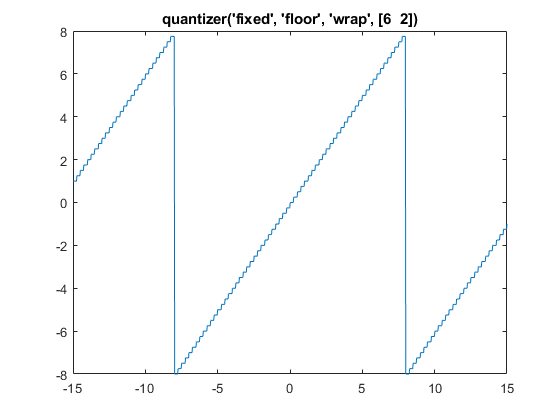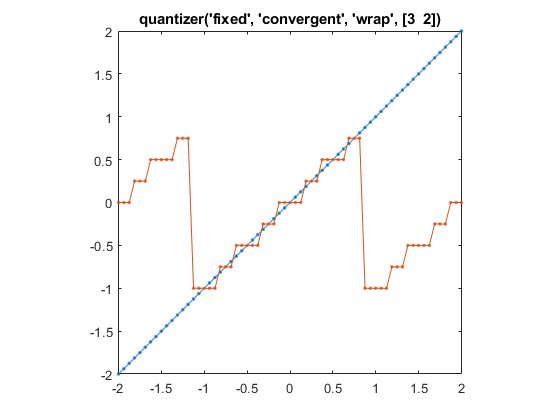# quantize

Quantize numeric data using `quantizer` object

## Syntax

``y = quantize(q,x)``
``[y1,y2,…] = quantize(q,x1,x2,…)``

## Description

example

````y = quantize(q,x)` uses the `quantizer` object `q` to quantize `x`.When `x` is a numeric array, each element of `x` is quantized. The output `y` is returned as a built-in double.When `x` is a cell array, each numeric element of the cell array is quantized. The fields of output `y` are returned as built-in doubles.When `x` is a structure, each numeric field of `x` is quantized. The fields of output `y` are returned as built-in doubles.`quantize` does not change nonnumeric elements or fields of `x`, nor does it issue warnings for nonnumeric values.The `quantizer` object states `max`, `min`, `noverflows`, `nunderflows`, and `noperations` are updated during the call to `quantize`, and running totals are kept until a call to `reset` is made.```

example

````[y1,y2,…] = quantize(q,x1,x2,…)` is equivalent to `y1 = quantize(q,x1), y2 = quantize(q,x2), …` and so forth.```

## Examples

collapse all

Use `quantize` to quantize data to a custom-precision floating-point type.

```x = linspace(-15,15,1000); q = quantizer('float','floor',[6 3]); range(q)```
```ans = 1×2 -14 14 ```
`y = quantize(q,x);`
```Warning: 68 overflow(s) occurred in the fi quantize operation. ```
`plot(x,y); title(tostring(q))`Use `quantize` to quantize data to a fixed-point type with a wordlength of 6 bits, a fraction length of 2 bits, round to floor, and wrap on overflow.

```x = linspace(-15,15,1000); q = quantizer('fixed','floor','wrap',[6 2])```
```q = DataMode = fixed RoundMode = floor OverflowMode = wrap Format = [6 2] ```
`range(q)`
```ans = 1×2 -8.0000 7.7500 ```
`y = quantize(q,x);`
```Warning: 468 overflow(s) occurred in the fi quantize operation. ```
`plot(x,y); title(tostring(q))`Use `quantize` to quantize data to a fixed-point type with a wordlength of 3 bits, a fraction length of 2 bits, convergent rounding, and wrap on overflow.

```q = quantizer('fixed','convergent','wrap',[3 2]); x = (-2:eps(q)/4:2)'; y = quantize(q,x);```
```Warning: 33 overflow(s) occurred in the fi quantize operation. ```
`plot(x,[x,y],'.-'); title(tostring(q)); axis square `## Input Arguments

collapse all

Data type properties to use for quantization, specified as a `quantizer` object.

Example: ```q = quantizer('fixed','ceil','saturate',[5 4]);```

Data to quantize, specified as a scalar, vector, matrix, multidimensional array, cell array, or structure.

• When `x` is a numeric array, each element of `x` is quantized.

• When `x` is a cell array, each numeric element of the cell array is quantized.

• When `x` is a structure, each numeric field of `x` is quantized.

`quantize` does not change nonnumeric elements or fields of `x`, nor does it issue warnings for nonnumeric values.

Data Types: `single` | `double` | `int8` | `int16` | `int32` | `int64` | `uint8` | `uint16` | `uint32` | `uint64` | `logical` | `struct` | `cell`
Complex Number Support: Yes

Data to quantize (as separate elements), specified as a scalar, vector, matrix, multidimensional array, cell array, or structure.

Data Types: `single` | `double` | `int8` | `int16` | `int32` | `int64` | `uint8` | `uint16` | `uint32` | `uint64` | `logical` | `struct` | `cell`
Complex Number Support: Yes

## Output Arguments

collapse all

Quantized data, returned as a scalar, vector, matrix, multidimensional array, cell array, or structure.

• When `x` is a numeric array, the output `y` is returned as a built-in double.

• When `x` is a cell array, the fields of output `y` are returned as built-in doubles.

• When `x` is a structure, the fields of output `y` are returned as built-in doubles.

Quantized data (as separate elements), returned as a scalar, vector, matrix, multidimensional array, cell array, or structure.

## Version History

Introduced in R2012b

expand all